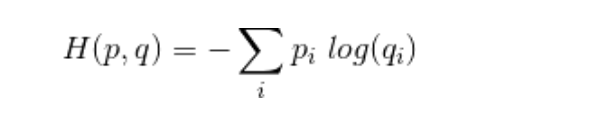# Unable to understand how pytorch calculates cross-entropy loss

Hi,
I came across this formula of how pytorch calculates cross-entropy loss-

loss(x, class) = -log(exp(x[class]) / (\sum_j exp(x[j])))
= -x[class] + log(\sum_j exp(x[j]))


Could anyone explain in more mathematical way which expression pytorch is using to calculate it as I have encountered only the following ways-where p is the target and q is the predicted

while another representation,I have seen is
Loss(Y_hat,Y) = -Ylog(Y_hat)

I mean, I am not able to understand the code which pytorch uses to calculate it.

The exp(x)/sum(exp(x)) defines the softmax.
Since p_i is the target, we avoid storing it in a one-hot encoded format (one 1, everything else zeros) and multiplying with it, as it’s wasteful. Instead we directly index the logit via x[class].

1 Like